# NCERT Exemplar Class 10 Maths Solutions for Chapter 1 - Real Numbers

## NCERT Exemplar Solutions Class 10 Maths Chapter 1 – Free PDF Download

NCERT Exemplar Class 10 Maths Chapter 1 Real Numbers are provided here for students to practice and prepare for the CBSE first and second term exam. The downloadable PDF is provided by our subject experts as per the CBSE guidelines (2021-2022). This material will help students to revise the syllabus of real numbers chapter and score good marks in the exam.

In this chapter, students will learn to solve problems based on topics like:

• Euclid’s Division Lemma
• The Fundamental Theorem of Arithmetic
• Revisiting Irrational Numbers
• Revisiting Rational Numbers and Their Decimal Expansions

Above given subtopics are widely explained in the NCERT Exemplar Class 10 Maths problems of Chapter 1 Class 10 is an important stage for students as they will be appearing for the board exams and the result they obtain will determine which subject to choose for further studies. Thus, in Class 10, students should develop a good understanding of each and every concept, especially in Maths. They should also sharpen their problem-solving skills if they want to succeed in the exams. Click here to get exemplars for all chapters.

There will be some amount of pressure of exam on students and in order to help them get better results, we are offering free NCERT Exemplar for Real Numbers here. The Exemplar has been designed by experts, based on CBSE syllabus to help students get a clear idea about two main topics – the fundamental theorem of arithmetic and Euclid’s division algorithm.

## Download PDF of NCERT Exemplar Class 10 Maths Chapter 1 Real Numbers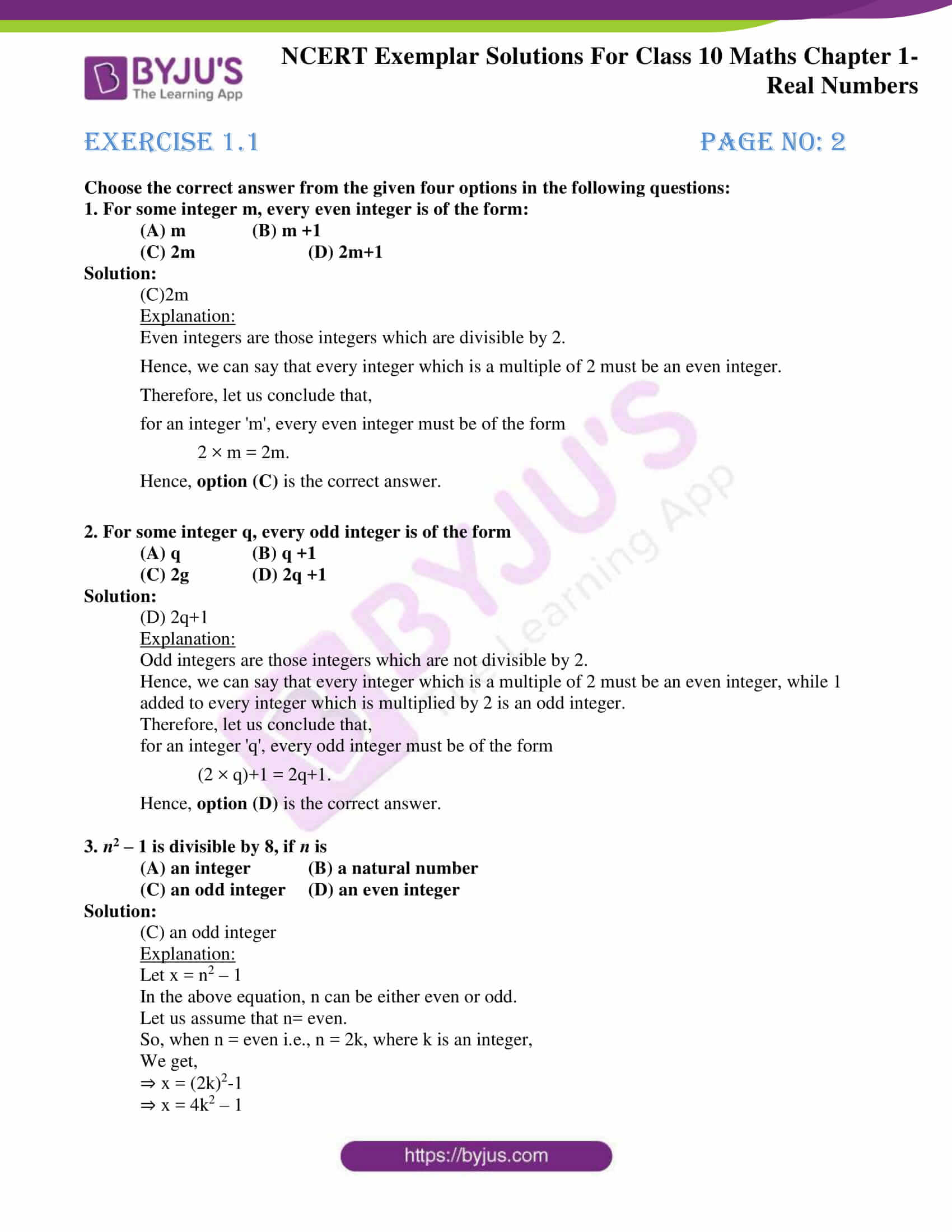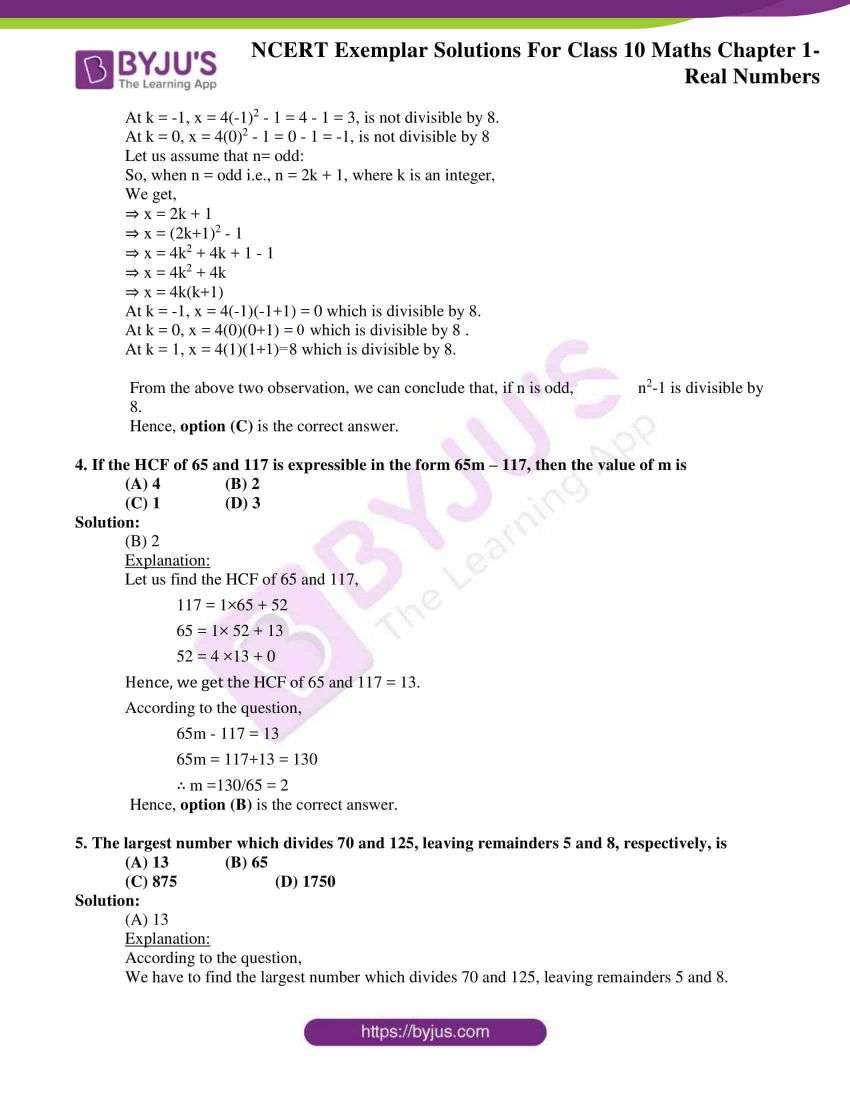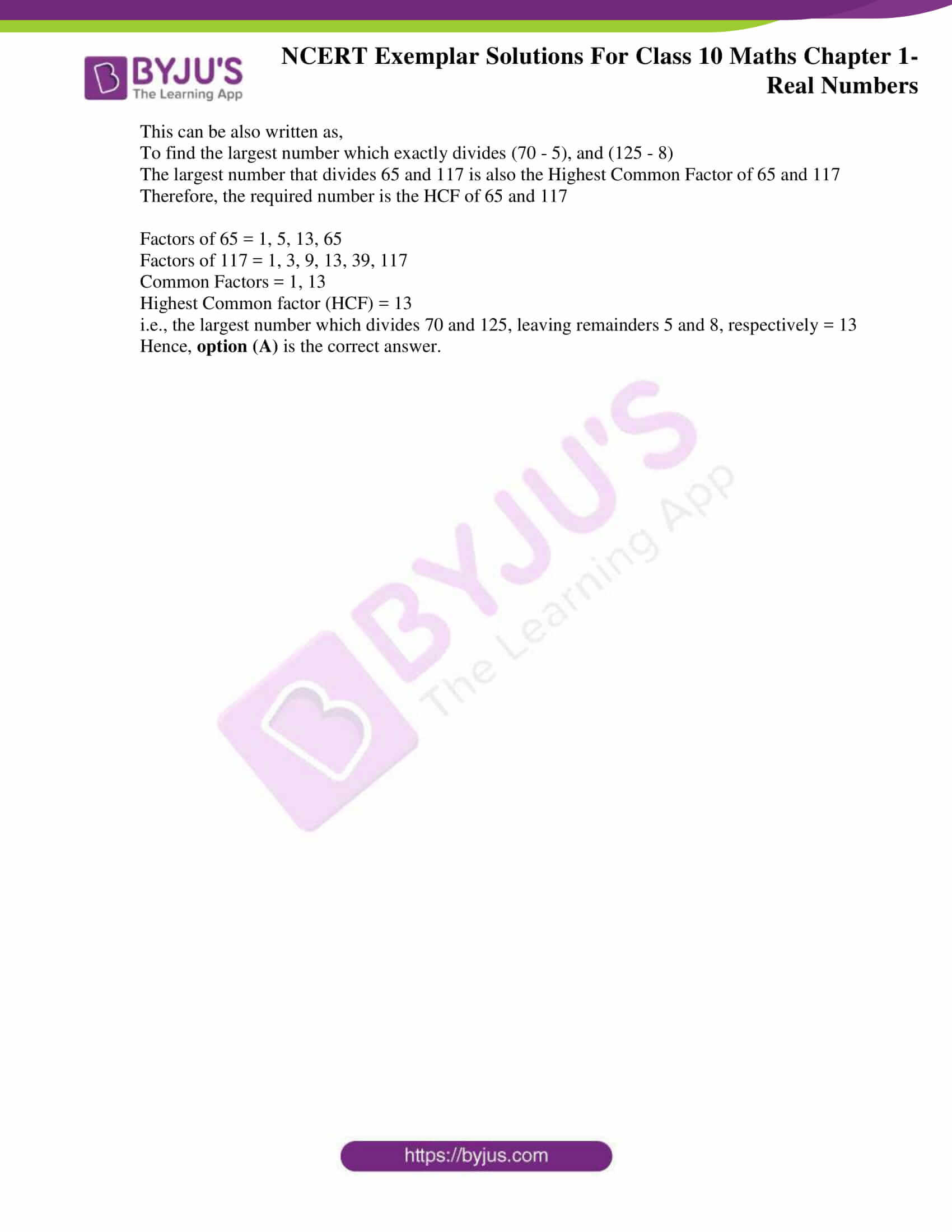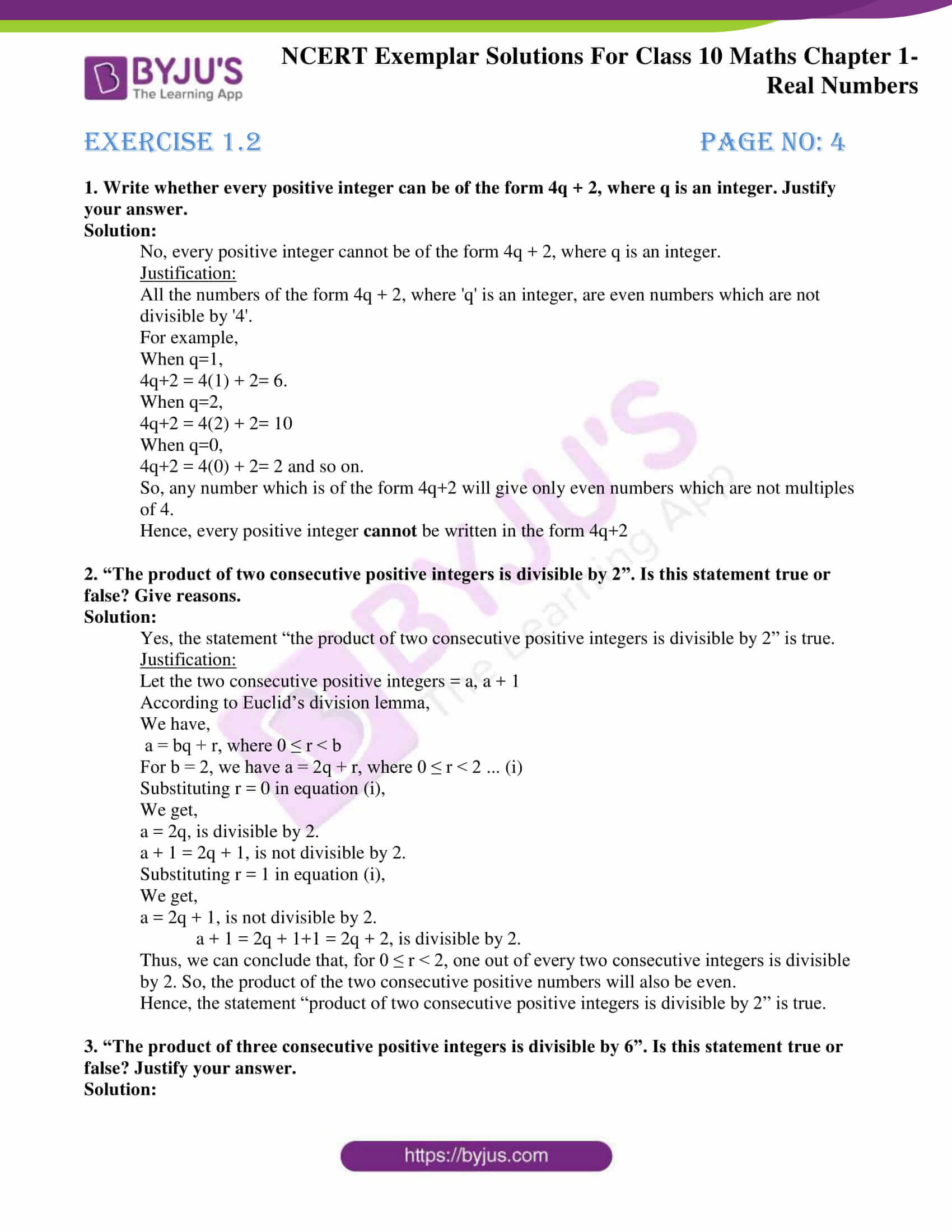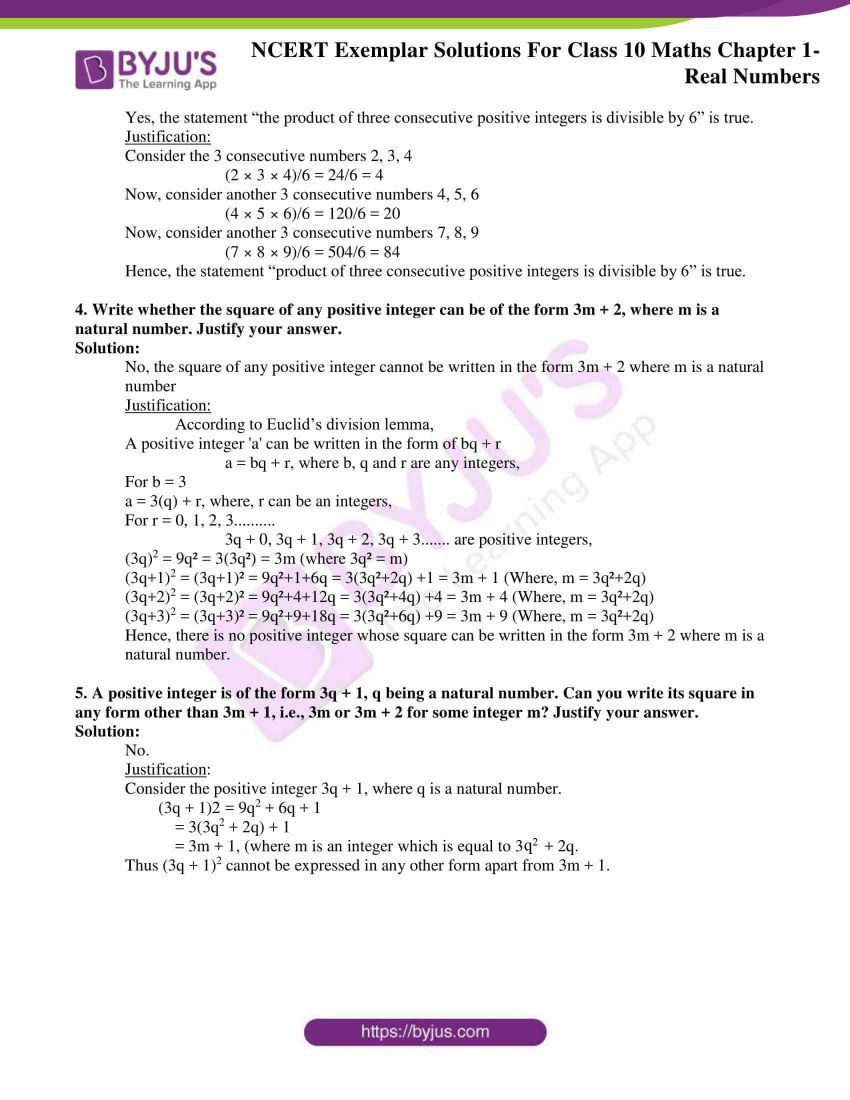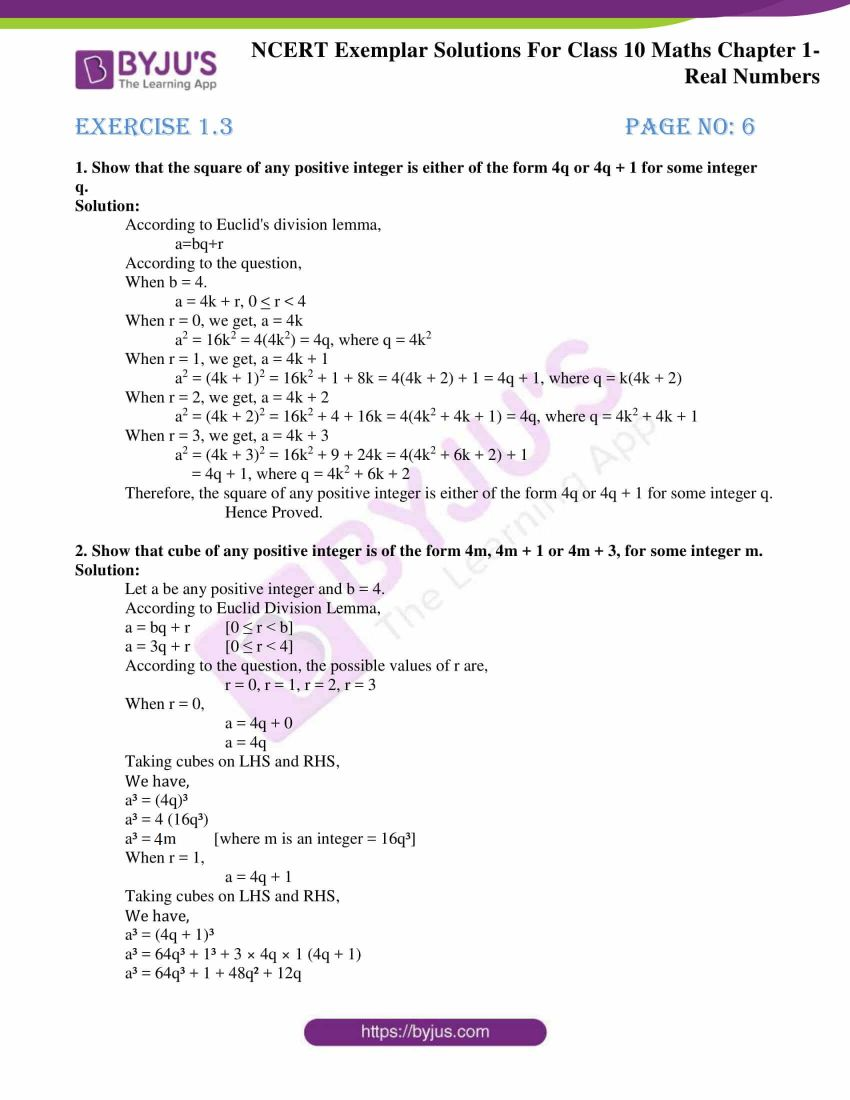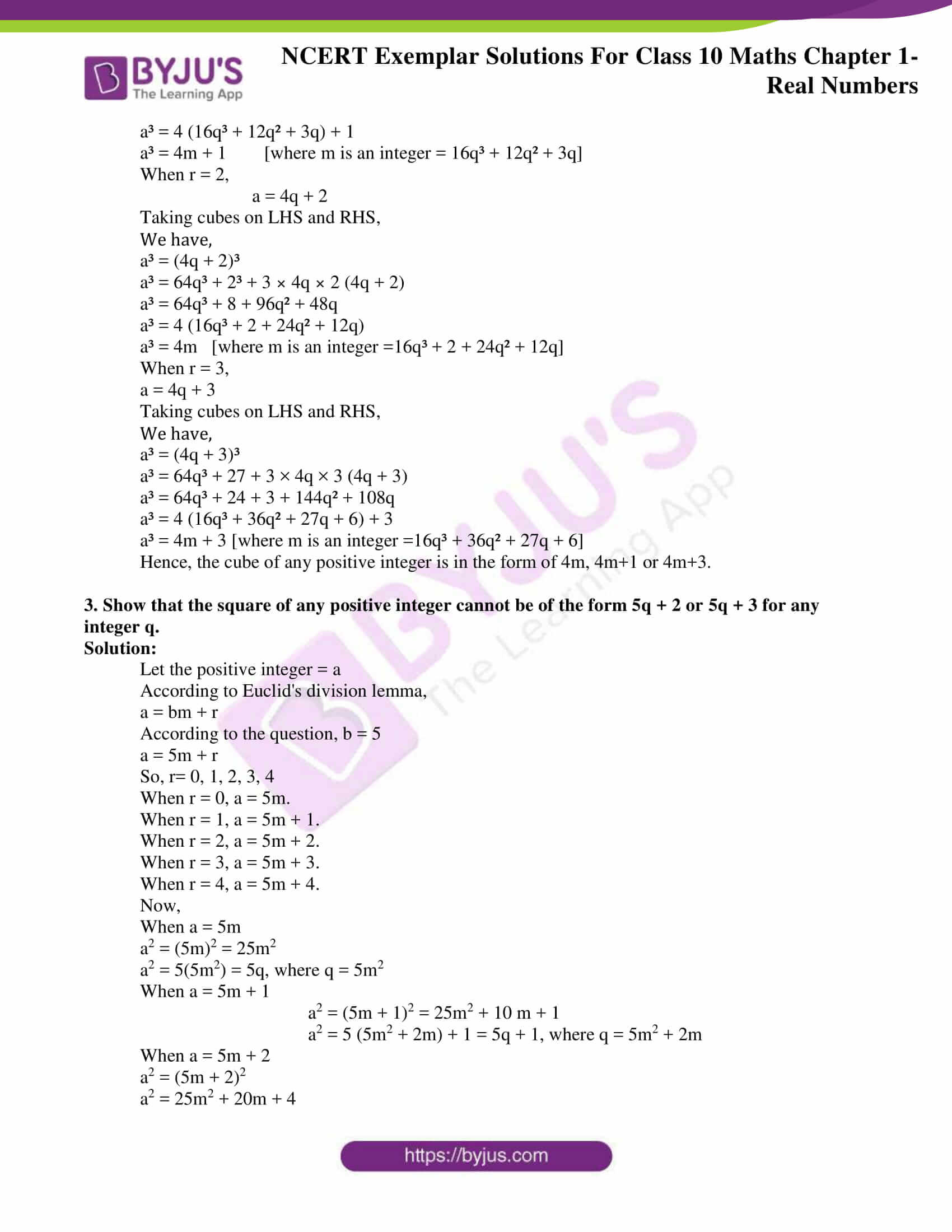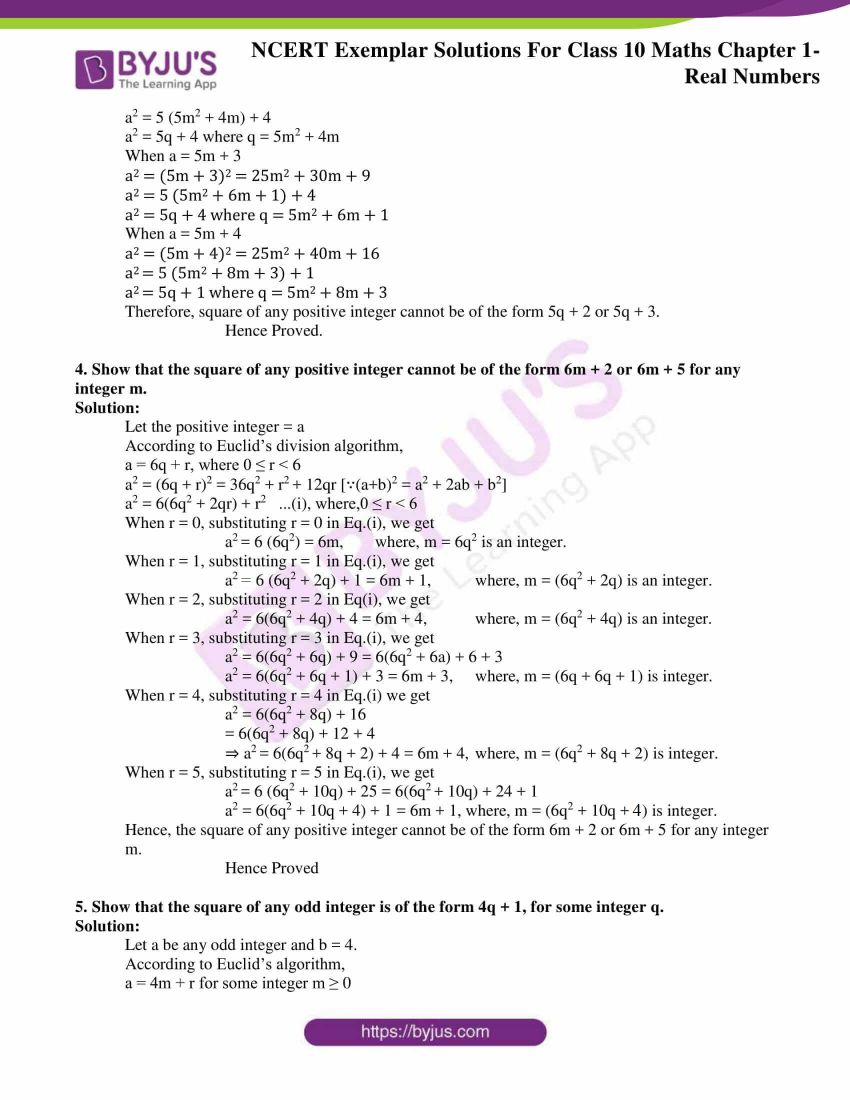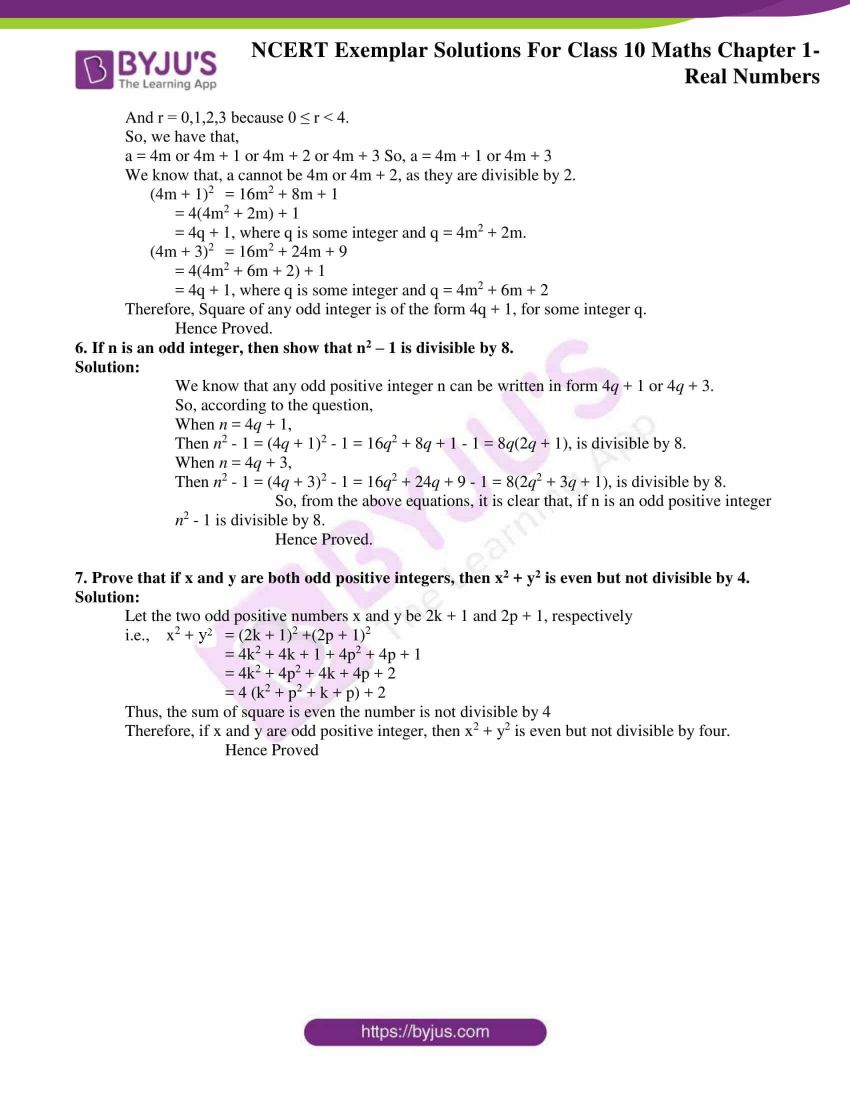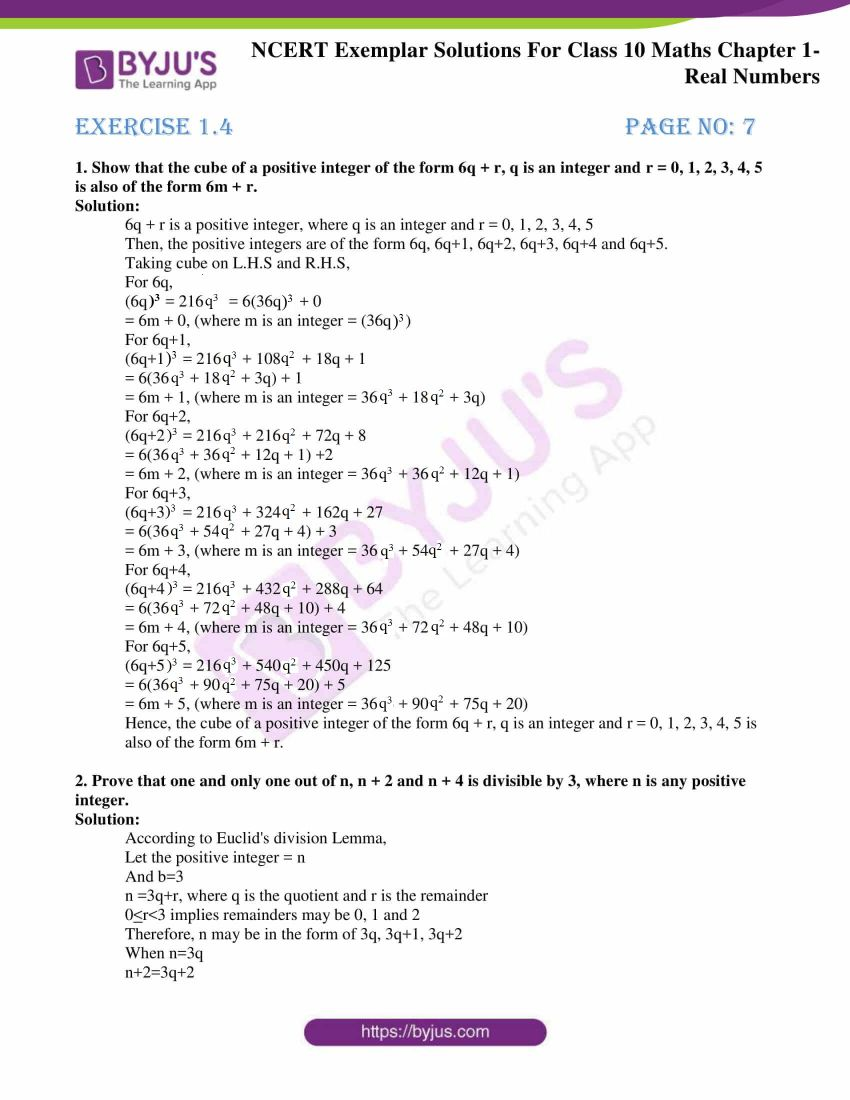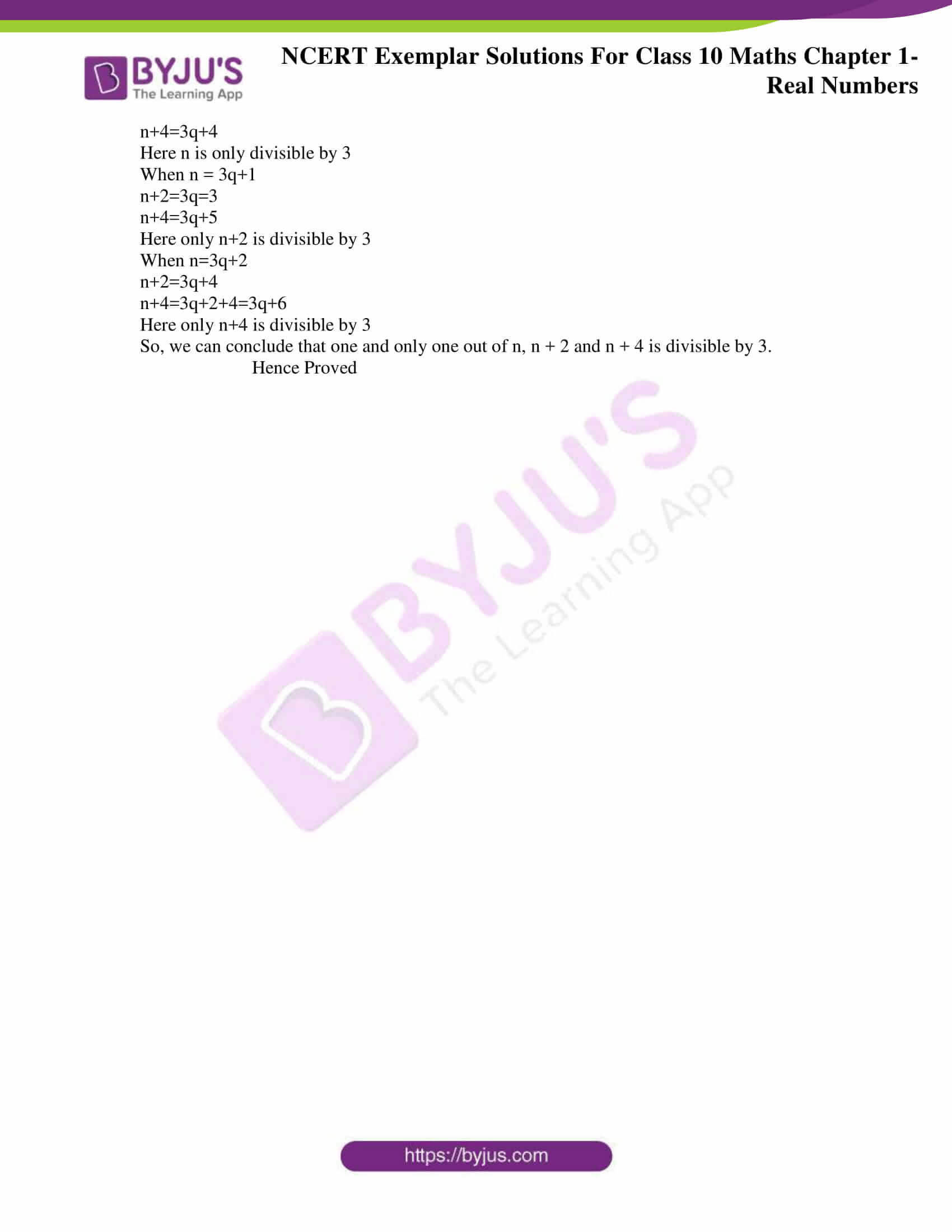## Real Numbers Exercise 1.1

Choose the correct answer from the given four options in the following questions:

1. For some integer m, every even integer is of the form:

(A) m (B) m +1

(C) 2m (D) 2m+1

Solution:

(C)2m

Explanation:

Even integers are those integers which are divisible by 2.

Hence, we can say that every integer which is a multiple of 2 must be an even integer.

Therefore, let us conclude that,

for an integer ‘m’, every even integer must be of the form

2 × m = 2m.

Hence, option (C) is the correct answer.

2. For some integer q, every odd integer is of the form

(A) q (B) q +1

(C) 2g (D) 2q +1

Solution:

(D) 2q+1

Explanation:

Odd integers are those integers which are not divisible by 2.

Hence, we can say that every integer which is a multiple of 2 must be an even integer, while 1 added to every integer which is multiplied by 2 is an odd integer.

Therefore, let us conclude that,

for an integer ‘q’, every odd integer must be of the form

(2 × q)+1 = 2q+1.

Hence, option (D) is the correct answer.

3. n2 – 1 is divisible by 8, if n is

(A) an integer (B) a natural number

(C) an odd integer (D) an even integer

Solution:

(C) an odd integer

Explanation:

Let x = n2 – 1

In the above equation, n can be either even or odd.

Let us assume that n= even.

So, when n = even i.e., n = 2k, where k is an integer,

We get,

⇒ x = (2k)2-1

⇒ x = 4k2 – 1

At k = -1, x = 4(-1)2 – 1 = 4 – 1 = 3, is not divisible by 8.

At k = 0, x = 4(0)2 – 1 = 0 – 1 = -1, is not divisible by 8

Let us assume that n= odd:

So, when n = odd i.e., n = 2k + 1, where k is an integer,

We get,

⇒ x = 2k + 1

⇒ x = (2k+1)2 – 1

⇒ x = 4k2 + 4k + 1 – 1

⇒ x = 4k2 + 4k

⇒ x = 4k(k+1)

At k = -1, x = 4(-1)(-1+1) = 0 which is divisible by 8.

At k = 0, x = 4(0)(0+1) = 0 which is divisible by 8 .

At k = 1, x = 4(1)(1+1) = 8 which is divisible by 8.

From the above two observation, we can conclude that, if n is odd, n2-1 is divisible by 8.

Hence, option (C) is the correct answer.

4. If the HCF of 65 and 117 is expressible in the form 65m – 117, then the value of m is

(A) 4 (B) 2

(C) 1 (D) 3

Solution:

(B) 2

Explanation:

Let us find the HCF of 65 and 117,

117 = 1×65 + 52

65 = 1× 52 + 13

52 = 4 ×13 + 0

Hence, we get the HCF of 65 and 117 = 13.

According to the question,

65m – 117 = 13

65m = 117+13 = 130

∴ m =130/65 = 2

Hence, option (B) is the correct answer.

5. The largest number which divides 70 and 125, leaving remainders 5 and 8, respectively, is

(A) 13 (B) 65

(C) 875 (D) 1750

Solution:

(A) 13

Explanation:

According to the question,

We have to find the largest number which divides 70 and 125, leaving remainders 5 and 8.

This can be also written as,

To find the largest number which exactly divides (70 – 5), and (125 – 8)

The largest number that divides 65 and 117 is also the Highest Common Factor of 65 and 117

Therefore, the required number is the HCF of 65 and 117

Factors of 65 = 1, 5, 13, 65

Factors of 117 = 1, 3, 9, 13, 39, 117

Common Factors = 1, 13

Highest Common factor (HCF) = 13

i.e., the largest number which divides 70 and 125, leaving remainders 5 and 8, respectively = 13

Hence, option (A) is the correct answer.

## Real Numbers Exercise 1.2

1. Write whether every positive integer can be of the form 4q + 2, where q is an integer. Justify your answer.

Solution:

No, every positive integer cannot be of the form 4q + 2, where q is an integer.

Justification:

All the numbers of the form 4q + 2, where ‘q’ is an integer, are even numbers which are not divisible by ‘4’.

For example,

When q=1,

4q+2 = 4(1) + 2= 6.

When q=2,

4q+2 = 4(2) + 2= 10

When q=0,

4q+2 = 4(0) + 2= 2 and so on.

So, any number which is of the form 4q+2 will give only even numbers which are not multiples of 4.

Hence, every positive integer cannot be written in the form 4q+2

2. “The product of two consecutive positive integers is divisible by 2”. Is this statement true or false? Give reasons.

Solution:

Yes, the statement “the product of two consecutive positive integers is divisible by 2” is true.

Justification:

Let the two consecutive positive integers = a, a + 1

According to Euclid’s division lemma,

We have,

a = bq + r, where 0 ≤ r < b

For b = 2, we have a = 2q + r, where 0 ≤ r < 2 … (i)

Substituting r = 0 in equation (i),

We get,

a = 2q, is divisible by 2.

a + 1 = 2q + 1, is not divisible by 2.

Substituting r = 1 in equation (i),

We get,

a = 2q + 1, is not divisible by 2.

a + 1 = 2q + 1+1 = 2q + 2, is divisible by 2.

Thus, we can conclude that, for 0 ≤ r < 2, one out of every two consecutive integers is divisible by 2. So, the product of the two consecutive positive numbers will also be even.

Hence, the statement “product of two consecutive positive integers is divisible by 2” is true.

3. “The product of three consecutive positive integers is divisible by 6”. Is this statement true or false? Justify your answer.

Solution:

Yes, the statement “the product of three consecutive positive integers is divisible by 6” is true.

Justification:

Consider the 3 consecutive numbers 2, 3, 4

(2 × 3 × 4)/6 = 24/6 = 4

Now, consider another 3 consecutive numbers 4, 5, 6

(4 × 5 × 6)/6 = 120/6 = 20

Now, consider another 3 consecutive numbers 7, 8, 9

(7 × 8 × 9)/6 = 504/6 = 84

Hence, the statement “product of three consecutive positive integers is divisible by 6” is true.

4. Write whether the square of any positive integer can be of the form 3m + 2, where m is a natural number. Justify your answer.

Solution:

No, the square of any positive integer cannot be written in the form 3m + 2 where m is a natural number

Justification:

According to Euclid’s division lemma,

A positive integer ‘a’ can be written in the form of bq + r

a = bq + r, where b, q and r are any integers,

For b = 3

a = 3(q) + r, where, r can be an integers,

For r = 0, 1, 2, 3……….

3q + 0, 3q + 1, 3q + 2, 3q + 3……. are positive integers,

(3q)2 = 9q² = 3(3q²) = 3m (where 3q² = m)

(3q+1)2 = (3q+1)² = 9q²+1+6q = 3(3q²+2q) +1 = 3m + 1 (Where, m = 3q²+2q)

(3q+2)2 = (3q+2)² = 9q²+4+12q = 3(3q²+4q) +4 = 3m + 4 (Where, m = 3q²+2q)

(3q+3)2 = (3q+3)² = 9q²+9+18q = 3(3q²+6q) +9 = 3m + 9 (Where, m = 3q²+2q)

Hence, there is no positive integer whose square can be written in the form 3m + 2 where m is a natural number.

5. A positive integer is of the form 3q + 1, q being a natural number. Can you write its square in any form other than 3m + 1, i.e., 3m or 3m + 2 for some integer m? Justify your answer.

Solution:

No.

Justification:

Consider the positive integer 3q + 1, where q is a natural number.

(3q + 1)2 = 9q2 + 6q + 1

= 3(3q2 + 2q) + 1

= 3m + 1, (where m is an integer which is equal to 3q2 + 2q.

Thus (3q + 1)2 cannot be expressed in any other form apart from 3m + 1.

## Real Numbers Exercise 1.3

1. Show that the square of any positive integer is either of the form 4q or 4q + 1 for some integer q.

Solution:

According to Euclid’s division lemma,

a=bq+r

According to the question,

When b = 4.

a = 4k + r, 0 < r < 4

When r = 0, we get, a = 4k

a2 = 16k2 = 4(4k2) = 4q, where q = 4k2

When r = 1, we get, a = 4k + 1

a2 = (4k + 1)2 = 16k2 + 1 + 8k = 4(4k + 2) + 1 = 4q + 1, where q = k(4k + 2)

When r = 2, we get, a = 4k + 2

a2 = (4k + 2)2 = 16k2 + 4 + 16k = 4(4k2 + 4k + 1) = 4q, where q = 4k2 + 4k + 1

When r = 3, we get, a = 4k + 3

a2 = (4k + 3)2 = 16k2 + 9 + 24k = 4(4k2 + 6k + 2) + 1

= 4q + 1, where q = 4k2 + 6k + 2

Therefore, the square of any positive integer is either of the form 4q or 4q + 1 for some integer q.

Hence Proved.

2. Show that cube of any positive integer is of the form 4m, 4m + 1 or 4m + 3, for some integer m.

Solution:

Let a be any positive integer and b = 4.

According to Euclid Division Lemma,

a = bq + r [0 ≤ r < b]

a = 3q + r [0 ≤ r < 4]

According to the question, the possible values of r are,

r = 0, r = 1, r = 2, r = 3

When r = 0,

a = 4q + 0

a = 4q

Taking cubes on LHS and RHS,

We have,

a³ = (4q)³

a³ = 4 (16q³)

a³ = 4m         [where m is an integer = 16q³]

When r = 1,

a = 4q + 1

Taking cubes on LHS and RHS,

We have,

a³ = (4q + 1)³

a³ = 64q³ + 1³ + 3 × 4q × 1 (4q + 1)

a³ = 64q³ + 1 + 48q² + 12q

a³ = 4 (16q³ + 12q² + 3q) + 1

a³ = 4m + 1        [where m is an integer = 16q³ + 12q² + 3q]

When r = 2,

a = 4q + 2

Taking cubes on LHS and RHS,

We have,

a³ = (4q + 2)³

a³ = 64q³ + 2³ + 3 × 4q × 2 (4q + 2)

a³ = 64q³ + 8 + 96q² + 48q

a³ = 4 (16q³ + 2 + 24q² + 12q)

a³ = 4m   [where m is an integer =16q³ + 2 + 24q² + 12q]

When r = 3,

a = 4q + 3

Taking cubes on LHS and RHS,

We have,

a³ = (4q + 3)³

a³ = 64q³ + 27 + 3 × 4q × 3 (4q + 3)

a³ = 64q³ + 24 + 3 + 144q² + 108q

a³ = 4 (16q³ + 36q² + 27q + 6) + 3

a³ = 4m + 3 [where m is an integer =16q³ + 36q² + 27q + 6]

Hence, the cube of any positive integer is in the form of 4m, 4m+1 or 4m+3.

3. Show that the square of any positive integer cannot be of the form 5q + 2 or 5q + 3 for any integer q.

Solution:

Let the positive integer = a

According to Euclid’s division lemma,

a = bm + r

According to the question, b = 5

a = 5m + r

So, r= 0, 1, 2, 3, 4

When r = 0, a = 5m.

When r = 1, a = 5m + 1.

When r = 2, a = 5m + 2.

When r = 3, a = 5m + 3.

When r = 4, a = 5m + 4.

Now,

When a = 5m

a2 = (5m)2 = 25m2

a2 = 5(5m2) = 5q, where q = 5m2

When a = 5m + 1

a2 = (5m + 1)2 = 25m2 + 10 m + 1

a2 = 5 (5m2 + 2m) + 1 = 5q + 1, where q = 5m2 + 2m

When a = 5m + 2

a2 = (5m + 2)2

a2 = 25m2 + 20m + 4

a2 = 5 (5m2 + 4m) + 4

a2 = 5q + 4 where q = 5m2 + 4m

When a = 5m + 3

a2 = (5m + 3)2 = 25m2 + 30m + 9

a2 = 5 (5m2 + 6m + 1) + 4

a2 = 5q + 4 where q = 5m2 + 6m + 1

When a = 5m + 4

a2 = (5m + 4)2 = 25m2 + 40m + 16

a= 5 (5m2 + 8m + 3) + 1

a= 5q + 1 where q = 5m2 + 8m + 3

Therefore, square of any positive integer cannot be of the form 5q + 2 or 5q + 3.

Hence Proved.

4. Show that the square of any positive integer cannot be of the form 6m + 2 or 6m + 5 for any integer m.

Solution:

Let the positive integer = a

According to Euclid’s division algorithm,

a = 6q + r, where 0 ≤ r < 6

a2 = (6q + r)2 = 36q2 + r+ 12qr [∵(a+b)2 = a2 + 2ab + b2]

a2 = 6(6q2 + 2qr) + r2   …(i), where,0 ≤ r < 6

When r = 0, substituting r = 0 in Eq.(i), we get

a= 6 (6q2) = 6m, where, m = 6q2 is an integer.

When r = 1, substituting r = 1 in Eq.(i), we get

a2 = 6 (6q2 + 2q) + 1 = 6m + 1, where, m = (6q2 + 2q) is an integer.

When r = 2, substituting r = 2 in Eq(i), we get

a2 = 6(6q2 + 4q) + 4 = 6m + 4, where, m = (6q2 + 4q) is an integer.

When r = 3, substituting r = 3 in Eq.(i), we get

a2 = 6(6q2 + 6q) + 9 = 6(6q2 + 6a) + 6 + 3

a2 = 6(6q2 + 6q + 1) + 3 = 6m + 3, where, m = (6q + 6q + 1) is integer.

When r = 4, substituting r = 4 in Eq.(i) we get

a2 = 6(6q2 + 8q) + 16

= 6(6q2 + 8q) + 12 + 4

⇒ a= 6(6q+ 8q + 2) + 4 = 6m + 4, where, m = (6q2 + 8q + 2) is integer.

When r = 5, substituting r = 5 in Eq.(i), we get

a= 6 (6q2 + 10q) + 25 = 6(6q+ 10q) + 24 + 1

a2 = 6(6q2 + 10q + 4) + 1 = 6m + 1, where, m = (6q2 + 10q + 4) is integer.

Hence, the square of any positive integer cannot be of the form 6m + 2 or 6m + 5 for any integer m.

Hence Proved

5. Show that the square of any odd integer is of the form 4q + 1, for some integer q.

Solution:

Let a be any odd integer and b = 4.

According to Euclid’s algorithm,

a = 4m + r for some integer m ≥ 0

And r = 0,1,2,3 because 0 ≤ r < 4.

So, we have that,

a = 4m or 4m + 1 or 4m + 2 or 4m + 3 So, a = 4m + 1 or 4m + 3

We know that, a cannot be 4m or 4m + 2, as they are divisible by 2.

(4m + 1)2 = 16m2 + 8m + 1

= 4(4m2 + 2m) + 1

= 4q + 1, where q is some integer and q = 4m2 + 2m.

(4m + 3)2 = 16m2 + 24m + 9

= 4(4m2 + 6m + 2) + 1

= 4q + 1, where q is some integer and q = 4m2 + 6m + 2

Therefore, Square of any odd integer is of the form 4q + 1, for some integer q.

Hence Proved.

6. If n is an odd integer, then show that n2 – 1 is divisible by 8.

Solution:

We know that any odd positive integer n can be written in form 4q + 1 or 4q + 3.

So, according to the question,

When n = 4q + 1,

Then n2 – 1 = (4q + 1)2 – 1 = 16q2 + 8q + 1 – 1 = 8q(2q + 1), is divisible by 8.

When n = 4q + 3,

Then n2 – 1 = (4q + 3)2 – 1 = 16q2 + 24q + 9 – 1 = 8(2q2 + 3q + 1), is divisible by 8.

So, from the above equations, it is clear that, if n is an odd positive integer

n2 – 1 is divisible by 8.

Hence Proved.

7. Prove that if x and y are both odd positive integers, then x2 + y2 is even but not divisible by 4.

Solution:

Let the two odd positive numbers x and y be 2k + 1 and 2p + 1, respectively

i.e., x2 + y2 = (2k + 1)2 +(2p + 1)2

= 4k2 + 4k + 1 + 4p2 + 4p + 1

= 4k2 + 4p2 + 4k + 4p + 2

= 4 (k2 + p2 + k + p) + 2

Thus, the sum of square is even the number is not divisible by 4

Therefore, if x and y are odd positive integer, then x2 + y2 is even but not divisible by four.

Hence Proved

## Real Numbers Exercise 1.4

1. Show that the cube of a positive integer of the form 6q + r, q is an integer and r = 0, 1, 2, 3, 4, 5 is also of the form 6m + r.

Solution:

6q + r is a positive integer, where q is an integer and r = 0, 1, 2, 3, 4, 5

Then, the positive integers are of form 6q, 6q+1, 6q+2, 6q+3, 6q+4 and 6q+5.

Taking cube on L.H.S and R.H.S,

For 6q,

(6q)³ = 216 q³ = 6(36q)³ + 0

= 6m + 0, (where m is an integer = (36q)³)

For 6q+1,

(6q+1)³ = 216q³ + 108q2 + 18q + 1

= 6(36q³ + 18q2 + 3q) + 1

= 6m + 1, (where m is an integer = 36q³ + 18q2 + 3q)

For 6q+2,

(6q+2)³ = 216q³ + 216q2 + 72q + 8

= 6(36q³ + 36q2 + 12q + 1) +2

= 6m + 2, (where m is an integer = 36q³ + 36q2 + 12q + 1)

For 6q+3,

(6q+3)³ = 216q³ + 324q2 + 162q + 27

= 6(36q³ + 54q2 + 27q + 4) + 3

= 6m + 3, (where m is an integer = 36q³ + 54q2 + 27q + 4)

For 6q+4,

(6q+4)³ = 216q³ + 432q2 + 288q + 64

= 6(36q³ + 72q2 + 48q + 10) + 4

= 6m + 4, (where m is an integer = 36q³ + 72q2 + 48q + 10)

For 6q+5,

(6q+5)³ = 216q³ + 540q2 + 450q + 125

= 6(36q³ + 90q2 + 75q + 20) + 5

= 6m + 5, (where m is an integer = 36q³ + 90q2 + 75q + 20)

Hence, the cube of a positive integer of the form 6q + r, q is an integer and r = 0, 1, 2, 3, 4, 5 is also of the form 6m + r.

2. Prove that one and only one out of n, n + 2 and n + 4 is divisible by 3, where n is any positive integer.

Solution:

According to Euclid’s division Lemma,

Let the positive integer = n

And b=3

n =3q+r, where q is the quotient and r is the remainder

0<r<3 implies remainders may be 0, 1 and 2

Therefore, n may be in the form of 3q, 3q+1, 3q+2

When n=3q

n+2=3q+2

n+4=3q+4

Here n is only divisible by 3

When n = 3q+1

n+2=3q=3

n+4=3q+5

Here only n+2 is divisible by 3

When n=3q+2

n+2=3q+4

n+4=3q+2+4=3q+6

Here only n+4 is divisible by 3

So, we can conclude that one and only one out of n, n + 2 and n + 4 is divisible by 3.

Hence Proved

The solved questions in exemplar Class 10 for Maths, will help students to prepare and score good marks in the final exam. Students are also provided with 10th Standard Maths NCERT Solutions, notes, question papers and other learning materials, online on our website for free. Also, solve previous year question papers and sample papers of Class 10 Maths subject, to know the question pattern and marking scheme for chapter Real Numbers.

Keep visiting BYJU’S and get all the updated learning materials for Class 10 Maths and Science subjects. Students are also recommended to download BYJU’S- The Learning App and get a personalized and modern learning experience with the help of educational videos explaining the concepts of topics like Real numbers.

## Frequently Asked Questions on NCERT Exemplar Solutions for Class 10 Maths Chapter 1

### What are the concepts explained in NCERT Exemplar Solutions for Class 10 Maths Chapter 1?

In this chapter, students will learn to solve problems based on topics like:
Euclid’s Division Lemma
The Fundamental Theorem of Arithmetic
Revisiting Irrational Numbers
Revisiting Rational Numbers and Their Decimal Expansions
The solutions to the exercise wise problems are solved by the set of highly experienced faculty members. The main objective of providing solutions is to help students score well in the Class 10 exams.

### What are the key features of NCERT Exemplar Solutions for Class 10 Maths Chapter 1?

The NCERT exercises are solved by the subject matter experts having vast knowledge about the important concepts. Each and every step is explained in easy and simple language to help students grasp the method of solving complex problems effortlessly. The solutions are solved in a stepwise manner based on the marks of the CBSE board.

### Is NCERT Exemplar Solutions for Class 10 Maths Chapter 1 enough to prepare for the board exams?

Chapter 1 covers all the important topics based on the latest CBSE guidelines. There are four exercises in this chapter providing the students with numerous problems to solve independently. The solutions are designed in such a way to boost the confidence in the minds of students before appearing for the board exams.